Ex 15.1

Chapter 15 Class 10 Probability
Serial order wise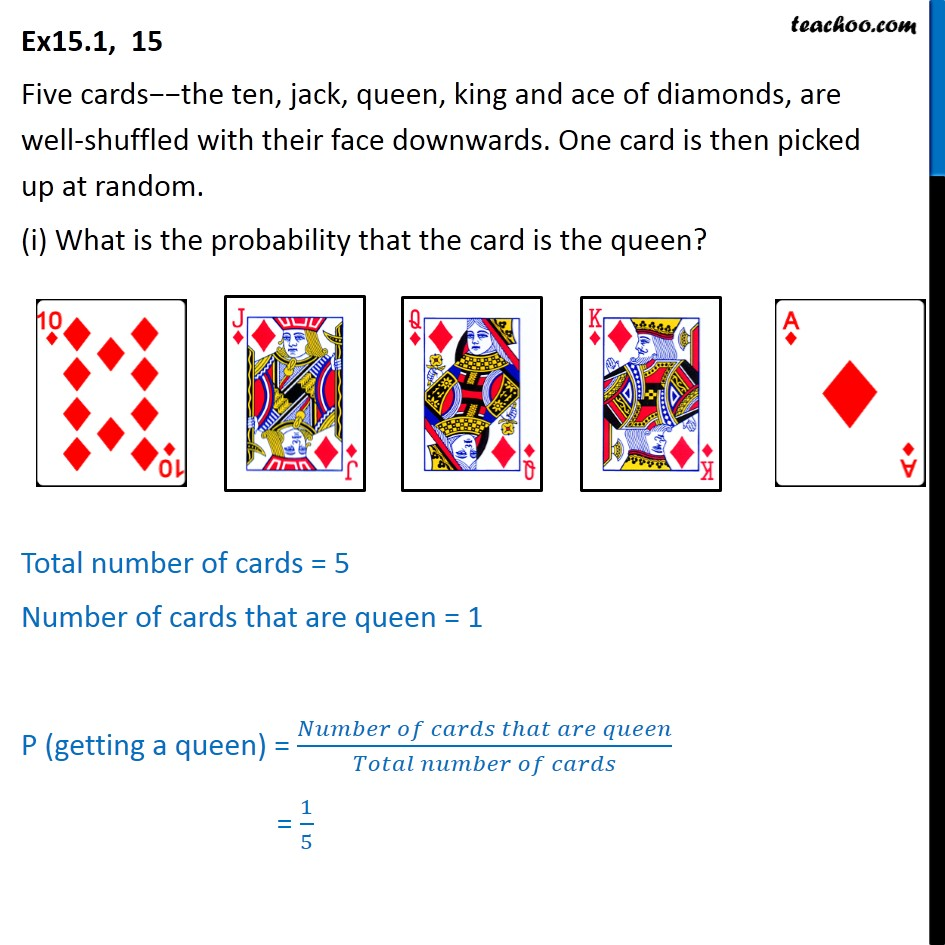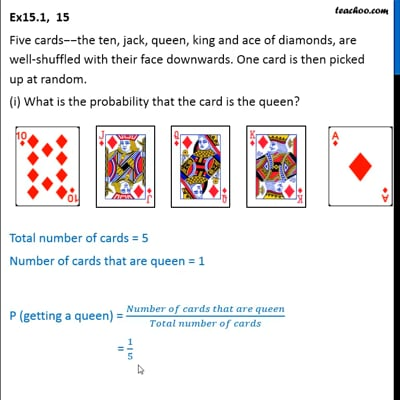This video is only available for Teachoo black users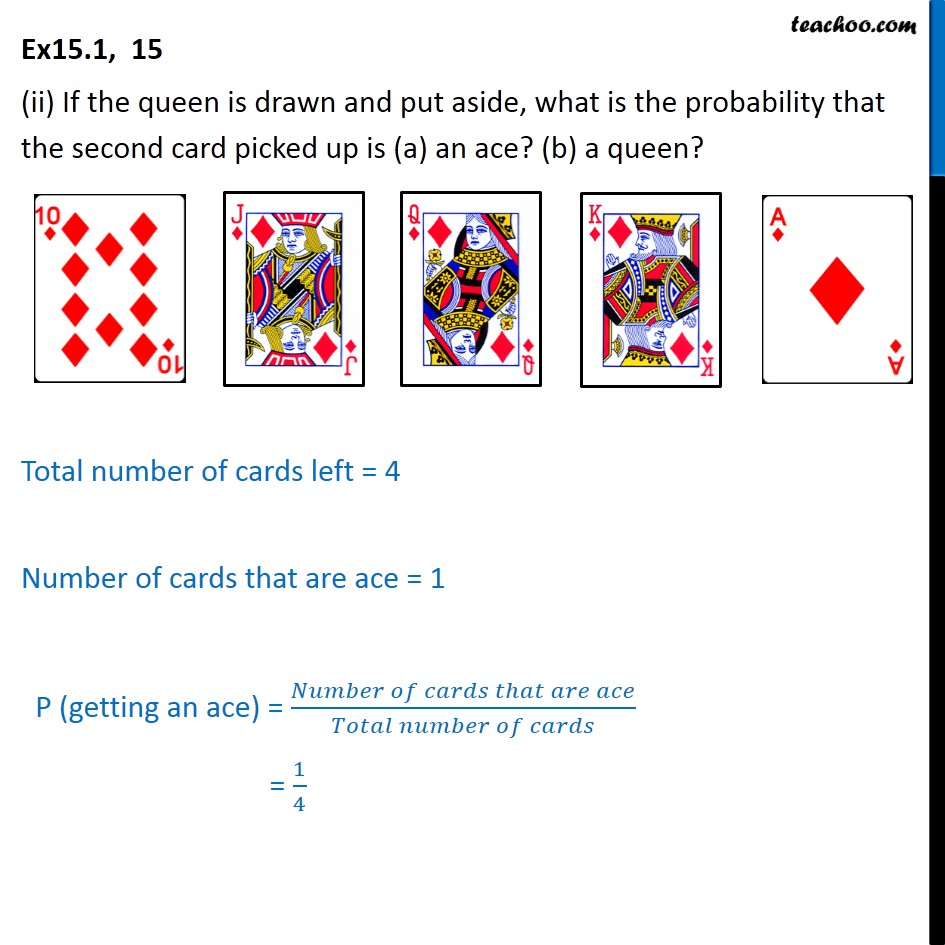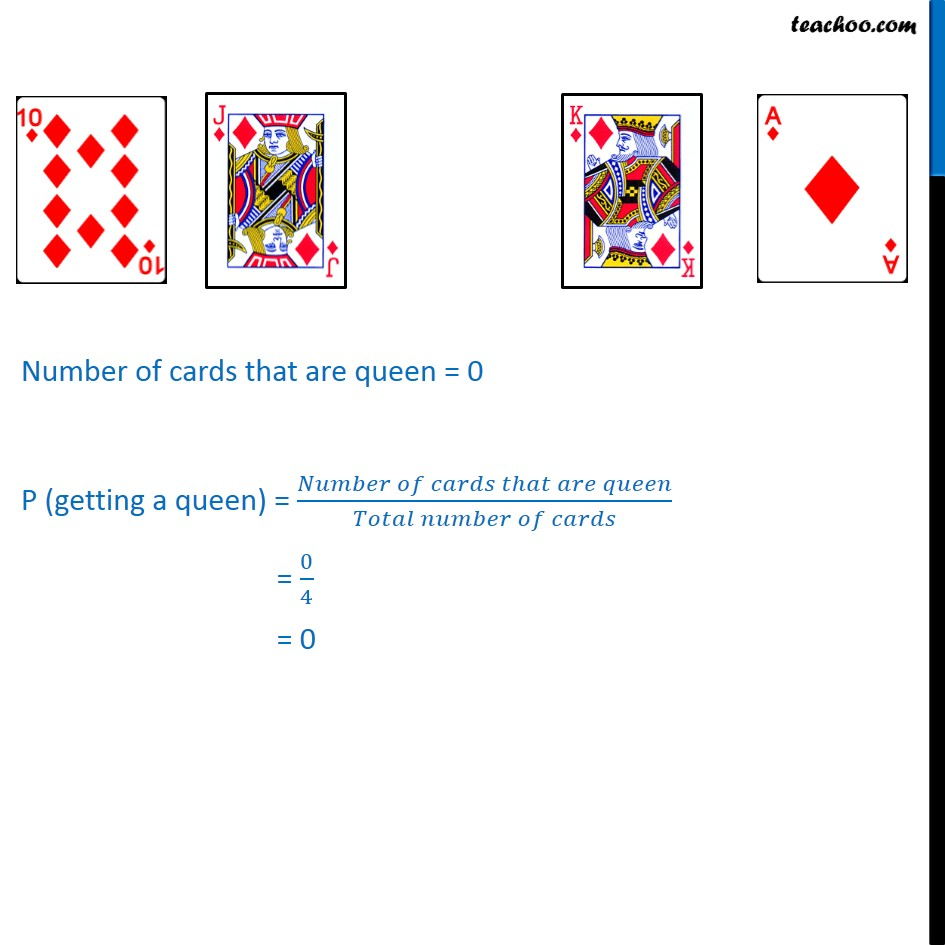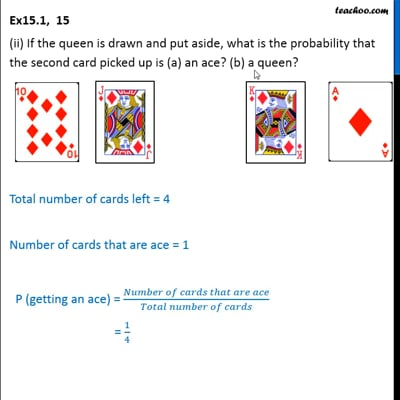This video is only available for Teachoo black users

Solve all your doubts with Teachoo Black (new monthly pack available now!)

### Transcript

Ex15.1, 15 Five cards−−the ten, jack, queen, king and ace of diamonds, are well-shuffled with their face downwards. One card is then picked up at random. (i) What is the probability that the card is the queen? Total number of cards = 5 Number of cards that are queen = 1 P (getting a queen) = (𝑁𝑢𝑚𝑏𝑒𝑟 𝑜𝑓 𝑐𝑎𝑟𝑑𝑠 𝑡ℎ𝑎𝑡 𝑎𝑟𝑒 𝑞𝑢𝑒𝑒𝑛)/(𝑇𝑜𝑡𝑎𝑙 𝑛𝑢𝑚𝑏𝑒𝑟 𝑜𝑓 𝑐𝑎𝑟𝑑𝑠) = 1/5 Ex15.1, 15 (ii) If the queen is drawn and put aside, what is the probability that the second card picked up is (a) an ace? (b) a queen? Total number of cards left = 4 Number of cards that are ace = 1 P (getting an ace) = (𝑁𝑢𝑚𝑏𝑒𝑟 𝑜𝑓 𝑐𝑎𝑟𝑑𝑠 𝑡ℎ𝑎𝑡 𝑎𝑟𝑒 𝑎𝑐𝑒)/(𝑇𝑜𝑡𝑎𝑙 𝑛𝑢𝑚𝑏𝑒𝑟 𝑜𝑓 𝑐𝑎𝑟𝑑𝑠) = 1/4 Number of cards that are queen = 0 P (getting a queen) = (𝑁𝑢𝑚𝑏𝑒𝑟 𝑜𝑓 𝑐𝑎𝑟𝑑𝑠 𝑡ℎ𝑎𝑡 𝑎𝑟𝑒 𝑞𝑢𝑒𝑒𝑛)/(𝑇𝑜𝑡𝑎𝑙 𝑛𝑢𝑚𝑏𝑒𝑟 𝑜𝑓 𝑐𝑎𝑟𝑑𝑠) = 0/4 = 0